# Braided category

braided monoidal category, quasi-tensor category

A generalization of the notion of tensor product of vector spaces, in which $\otimes$ is associative only up to isomorphism and the transposition isomorphism $\otimes \rightarrow \otimes ^ { \text{op} }$ need not square to the identity.

The full definition is: a category $\mathcal{C}$ of objects $V$, $W$, $Z$, etc., equipped with a functor $\otimes : \mathcal{C} \times \mathcal{C} \rightarrow \mathcal{C}$, and a collection of functorial isomorphisms

\begin{equation*} \Phi _ { V , W , Z } : ( V \bigotimes W ) \bigotimes Z \rightarrow V \bigotimes ( W \bigotimes Z ) \end{equation*}

(called the associator) between any three objects and

\begin{equation*} \Psi _ { V , W } : V \bigotimes W \rightarrow W \bigotimes V \end{equation*}

(called the braiding) between any two objects. "Functorial" means that these isomorphisms commute with any morphisms between objects. Thus,

\begin{equation*} ( \phi \bigotimes \text { id } ) \Psi _ { V , W } = \Psi _ { V , Z } ( \text { id } \bigotimes \phi ) , \forall \phi : W \rightarrow Z, \end{equation*}

if the morphism is applied before or after the braiding. Similarly for functoriality in the other arguments of $\Psi$ and in the arguments of $\Phi$. The precise definition is that $\Phi : ( \otimes ) \otimes \rightarrow \otimes ( \otimes )$ and $\Psi : \otimes \rightarrow \otimes ^ { \text{ op} }$ are natural equivalences, where $( \otimes ) \otimes :\mathcal C \times \mathcal C \times \mathcal C \rightarrow \mathcal C$ is the functor sending $( V , W , Z )$ to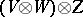, etc., and $\otimes ^{\operatorname{op}} : \mathcal{C} \times \mathcal{C} \rightarrow \mathcal{C}$ is the functor sending $( V , W )$ to $W \otimes V$.

In addition, these functors are required to be coherent. The coherence for $\Phi$ is the pentagon identity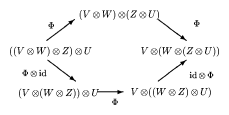Figure: b120420a

It says that the two ways to reverse the bracketings as shown coincide. MacLane's coherence theorem says that then all other routes between two bracketed tensor products also coincide. In effect, this means that one may generalize constructions in linear algebra exactly as if $\otimes$ were strictly associative, dropping brackets. Afterwards one may add brackets, for example with brackets accumulating to the left, and then insert applications of $\Phi$ as needed for the desired compositions to make sense; all different ways to do this will yield the same net result.

One also requires a unit object $1$ and an associated collection of functorial isomorphisms $r _{V} : V \rightarrow V \otimes \underline { 1 }$ and $l _ { V } : V \rightarrow \underline { 1 } \otimes V$ obeying a triangle coherence identity

\begin{equation*} \operatorname { id} \bigotimes r _ { W } = \Phi _ { V , 1 , W } \circ ( l _ { V } \bigotimes \text { id } ). \end{equation*}

This structure $( \mathcal C , \otimes , \Phi , \underline { 1 } , l , r )$ is called a monoidal category (see also Triple).

The coherence conditions for the additional structure $\Psi$ of a braided category are the so-called hexagon identities

\begin{equation*} \Psi _ { V \bigotimes W , Z } = \Psi _ { V , Z } \circ \Psi _ { W , Z }, \end{equation*}

\begin{equation*} \Psi _ { V , W \bigotimes Z } = \Psi _ { V ,\, Z } \circ \Psi _ { V , W } . \end{equation*}

Here and below, the bracketings and $\Phi$ needed to make sense of these identities are omitted. (When they are inserted, each identity corresponds to a diagram with six arrows.) One can show that compatibility of $\Psi$ with $1$ is then automatic.

Notice that although $\Psi$ generalizes the concept of transposition of vector spaces, one does not demand that $\Psi _ { V , W } = \Psi _ { W , V } ^ { - 1 }$. If this does hold for all $V$, $W$, then a symmetric monoidal category or tensor category is obtained. In this case the two hexagon identities are equivalent and ensure that all ways to go

\begin{equation*} V _ { 1 } \bigotimes \ldots \bigotimes V _ { n } \rightarrow V _ { \sigma ( 1 ) } \bigotimes \ldots \bigotimes V _ { \sigma ( n ) } \end{equation*}

($\sigma$ a permutation) by composing $\Psi$ (and $\Phi$) yield the same result. In particular, there is an action of the symmetric group $S _ { n }$ in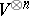for any object $V$.

For a general braided category there is a similar result in terms of braids. To explain this, the following notational device is used: instead of writing the morphisms $\Psi _ { V , W }$ and $\Psi _ { W , V } ^ { - 1 }$ in the usual way as arrows, one writes them as braids and assumes that they point downwards:Figure: b120420b

One writes another morphism $\phi : W \rightarrow Z$, say, as a node on a strand connecting $W$ to $Z$ and assumed to be pointing downwards. Functoriality says that morphisms can be pulled through braid crossings asFigure: b120420c

Similarly for morphisms on the other strand. When the tensor product of several morphisms is applied, one writes their strands separately side by side, connecting the relevant objects in the tensor product. On the other hand, one is free to group some of the objects in the tensor product together as a single object and represent morphisms to and from it by a single strand. This notation is consistent precisely because of the hexagon conditions, which become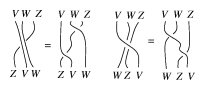Figure: b120420d

The doubled strands on the left-hand side could be replaced by single strands for the composite morphisms.

The coherence theorem for braided categories then asserts that different routes between tensor product expressions by repeated applications of $\Phi$, $\Psi$ and their inverses compose to the same morphism if the corresponding braids are the same. In particular, for any object $V$ there is an action of the pure braid group $B _ { n }$ on. The former can be presented as

\begin{equation*} b _ { i } b _ { i + 1} b _ { i } = b _ { i + 1} b _ { i } b _ { i } + 1 , b _ { i } b _ { j } = b _ { j } b _ { i } , \quad | i - j | \geq 2, \end{equation*}

where $b _ { i }$ is represented by $\Psi$ acting in the $( i , i + 1 )$th copies of $V$. The representation usually has a kernel and $B _ { n }$ modulo this kernel is the Hecke algebra associated to an object in a braided category.

Finally, an object $V$ in a braided category is rigid if there are another object $V ^ { * }$ and morphisms $\operatorname{ev} _ { V } : V ^ { * } \otimes V \rightarrow \underline { 1 }$ and $\operatorname{coev}_V : \underline { 1 } \rightarrow V \otimes V ^ { * }$ such that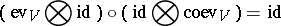(suppressing $\Phi$, $l$, $r$). Here, $V ^ { * }$ is called a left dual of $V$ and is unique up to isomorphism (there is a similar notion of right dual). $\mathcal{C}$ is called rigid if every object has such duals. Using diagrammatic notation one writesFigure: b120420e

and the above axioms become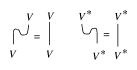Figure: b120420f

Note that in a rigid braided category, morphisms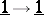look like knots (cf. also Knot theory; Braid theory). Every knot presented on paper is in fact the closure of some braid, so with a little more structure (notably, both left and right duals) one can arrange that every oriented knot can be read from top to bottom as a morphism. Fixing an object $V$,can be read as a left or right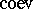andcan be read as a left or right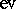(in accordance with orientation). One can read braid crossings as $\Psi$ or $\Psi ^ { - 1 }$. The result is not quite a knot invariant but can usually be adjusted to become one.

## Examples.

Some standard examples of braided categories are provided by the following constructions over a ground field $k$. Its invertible elements are denoted $k ^ { * }$.

1) Fix $q \in k$, an $n$th root of unity. The category of anyspaces $\operatorname{Vec}_n$ consists of $\mathbf{Z} / n \mathbf{Z}$-graded vector spaces $V = \oplus _ { i = 0 } ^ { n - 1 } V _ { i }$ (where the elements of $V _ { i }$ have degree $i$). Morphisms are degree-preserving linear mappings. One takes the associator trivial and the braiding

\begin{equation*} \Psi _ { V , W } ( v \bigotimes w ) = q ^ { |v| | w | } w \bigotimes v \end{equation*}

for $v \in V$ and $w \in W$ of degree $v$, $w$, respectively.

Clearly, $\operatorname{Vec}_{1}$ is the usual category of vector spaces, while $\text{Vec}_2$ is the category of linear superspaces or ${\bf Z} / 2 {\bf Z}$-graded vector spaces. The category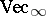of $\mathbf{Z}$-graded spaces is braided similarly for any $q \in k ^ { * }$.

A similar construction works for grading by any Abelian group $G$ equipped with a bicharacter $\beta : G \times G \rightarrow k ^ { * }$ (a function multiplicative in each argument) and braiding

\begin{equation*} \Psi _ { V , W } ( v \bigotimes w ) = \beta ( | v | , | w | ) w \bigotimes v \end{equation*}

on elements of degree $| v | , | w | \in G$.

2) If $G$ is a group and $\phi : G \times G \times G \rightarrow k ^ { * }$ a group $3$-cocycle, then the category of $G$-graded spaces is monoidal with associator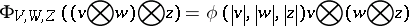on elements of degree $| v | , | w | , | z | \in G$. If $G$ is Abelian and equipped with a quasi-bicharacter with respect to $\phi$, then the category is braided.

For example, the octonion algebra lives naturally in such a category with $G = \mathbf{Z} _ { 2 } \times \mathbf{Z} _ { 2 } \times \mathbf{Z} _ { 2 }$ and $\phi$ a certain coboundary.

3) The category of finite-dimensional representations of the quantum enveloping algebras (cf. also Quantum groups; Universal enveloping algebra) $U _ { q } ( \mathfrak { g } )$ associated to a semi-simple Lie algebra $\frak g$. Also, certain classes of infinite-dimensional representations. Associated to the standard representation of $U _ { q } ( \operatorname{sl} _ { 2 } )$ is the standard Hecke algebra defined by the additional relation $( b _ { i } - q ) ( b _ { i } + q ^ { - 1 } ) = 0$. The knot invariant associated to this same representation is the Jones knot polynomial.

In an algebraic formulation, if $H$, $\mathcal{R} \in H \otimes H$, is a quasi-triangular Hopf algebra, then its category $\square _ { H } \cal M$ of representations is braided with $\Psi = \tau \circ \mathcal{R}$, where one first acts with the quasi-triangular structure $\mathcal{R} \in H \otimes H$ and then applies the usual transposition mapping $\tau$.

4) The category of co-modules under the matrix quantum groups (cf. Quantum groups) $G_q$. For example, the quantum plane generated by $x , y$ modulo the relations $y x = q x y$ is covariant under $\operatorname{SL} _ { q } ( 2 )$ and hence lives as an algebra in its braided category of co-modules. Likewise, the category of co-modules under a quantum matrix bi-algebra $A ( R )$ associated to an invertible solution $R$ of the Yang–Baxter equation is braided.

In a general algebraic formulation, if $H$, $\mathcal{R} : H \otimes H \rightarrow k$, is a dual (or co-) quasi-triangular Hopf algebra, then its category of co-modules is braided by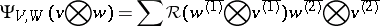where $\sum _ { V } v ^ { \overline{( 1 ) }} \otimes v ^ { \overline{( 2 ) } }$ denotes the result of the co-action $V \rightarrow H \otimes V$.

5) If $H$ is any Hopf algebra with invertible antipode, then its category $\square _ { H } ^ { H } \mathcal{M}$ of crossed modules (also called Drinfel'd–Radford–Yetter modules) is braided. Here, objects are vector spaces $V$ which are both modules and co-modules under $H$, the two being compatible in the sense

\begin{equation*} \sum h_{ ( 1 )} v ^ { \overline{( 1 )} } \bigotimes h_{ ( 2 )} \rhd v ^ { \overline{( 2 )} } = \end{equation*}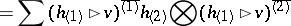for $h \in H$ and $v \in V$, where $\triangleright$ denotes the action and $\sum h _ { ( 1 ) } \otimes h _ { ( 2 ) }$ denotes the co-product. The braiding is

\begin{equation*} \Psi _ { V , W } ( v \bigotimes w ) = \sum v ^ { \overline{( 1 )} } \rhd w \bigotimes v ^ { \overline{( 2 )} }. \end{equation*}

For example, if $\frak g$ is any Lie algebra and $U ( \mathfrak { g } )$ is its enveloping algebra (cf. also Universal enveloping algebra), then $V = k 1 \oplus \mathfrak g \subset U ( \mathfrak g )$ is a crossed module by the adjoint action and the co-product of $U ( \mathfrak { g } )$. The braiding induced on it is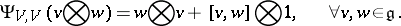6) Let $\mathcal{C}$ be any monoidal category. Then its representation-theoretic dual category $\mathcal{C} ^ { \circ }$ (also called the double $D ( \mathcal{C} )$ or centre $Z (\cal C )$) is a braided category. Objects are pairs $( V , \lambda )$ where $V$ is an object of $\mathcal{C}$ and $\lambda _ { W } : V \otimes W \rightarrow W \otimes V$ is a collection of functorial isomorphisms representing the $\otimes$ of $\mathcal{C}$ in the sense

\begin{equation*} \lambda _ { \underline{1} } = \operatorname {id} , \lambda _ { W \bigotimes Z} = \lambda_{Z} \circ \lambda _ { W } \end{equation*}

($\Phi$, $l$, $r$ suppressed in this notation). The braiding is $\Psi _ { ( V , \lambda ) , ( W , \mu ) } = \lambda _ { W }$.

These constructions are in roughly increasing order of generality. Thus, the categorical dual or double construction applied to the category of $H$-modules yields the category of crossed modules $\square _ { H } ^ { H } \mathcal{M}$. These, in turn, are an elementary reformulation (and thereby a slight generalization to infinite-dimensional $H$) of the category of $D ( H )$-modules, where $D ( H )$ is the quantum double quasi-triangular Hopf algebra associated to any finite-dimensional Hopf algebra $H$. Meanwhile, a bicharacter on an Abelian group extends by linearity to a dual-quasi-triangular structure on the corresponding group algebra.

How to Cite This Entry:
Braided category. Encyclopedia of Mathematics. URL: http://encyclopediaofmath.org/index.php?title=Braided_category&oldid=50577
This article was adapted from an original article by S. Majid (originator), which appeared in Encyclopedia of Mathematics - ISBN 1402006098. See original article